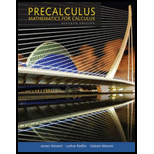## Solutions for Precalculus: Mathematics for Calculus (Standalone Book)

### Book Details

This bestselling author team explains concepts simply and clearly, without glossing over difficult points. Problem solving and mathematical modeling are introduced early and reinforced throughout, providing students with a solid foundation in the principles of mathematical thinking. Comprehensive and evenly paced, the book provides complete coverage of the function concept, and integrates a significant amount of graphing calculator material to help students develop insight into mathematical ideas. The authors' attention to detail and clarity--the same as found in James Stewart's market-leading Calculus text--is what makes this text the proven market leader.

## Sample Solutions for this Textbook

We offer sample solutions for Precalculus: Mathematics for Calculus (Standalone Book) homework problems. See examples below:

## More Editions of This Book

Corresponding editions of this textbook are also available below:

PRECALCULUS
7th Edition
ISBN: 9781337884440
Precalculus: Mathematics for Calculus
7th Edition
ISBN: 9781305115309
WebAssign Printed Access Card for Stewart/Redlin/Watson's Precalculus, Enhanced Edition, 7th Edition, Single-Term
7th Edition
ISBN: 9781337652360
Rutgers University Precalculus Mathematics for Calculus 640: 111/112/115 + Enhanced Web Assign Printed Access Card BNDL
7th Edition
ISBN: 9781305743847
Student Solutions Manual for Stewart/Redlin/Watson's Precalculus: Mathematics for Calculus, 7th
7th Edition
ISBN: 9781305253612
PRECALCULUS: MATHEMATICS FOR CALCULUS
7th Edition
ISBN: 9781305718906
PRECALCULUS LL + WEBASSIGN/COREQUISITE
7th Edition
ISBN: 9780357293270
Precalculus
7th Edition
ISBN: 9781305761049
EBK PRECALCULUS: MATHEMATICS FOR CALCUL
7th Edition
ISBN: 9781337431125
Precalculus - Mathematics for Calculus - Seventh Edition
7th Edition
ISBN: 9781305750463
Bundle: Precalculus: Mathematics for Calculus, 7th + WebAssign Printed Access Card for Stewart/Redlin/Watson's Precalculus, Enhanced Edition, 7th Edition, Single-Term
7th Edition
ISBN: 9781305701618
Precalculus - A Custom Text for UNLV
6th Edition
ISBN: 9781133150572
Precalculus: Mathematics for Calculus - 6th Edition
6th Edition
ISBN: 9780840068071
EBK PRECALCULUS: MATHEMATICS FOR CALCUL
6th Edition
ISBN: 9781133715047
EBK PRECALCULUS: MATHEMATICS FOR CALCUL
6th Edition
ISBN: 8220100466571
Precalculus, Enhanced Webassign Edition (Book Only)
6th Edition
ISBN: 9781133954750
Precalculus: Mathematics for Calculus, 5th Edition
5th Edition
ISBN: 9780534492779
Precalculus: Mathematics For Calculus, Enhanced Review Edition, 5th Edition
5th Edition
ISBN: 9780495557500